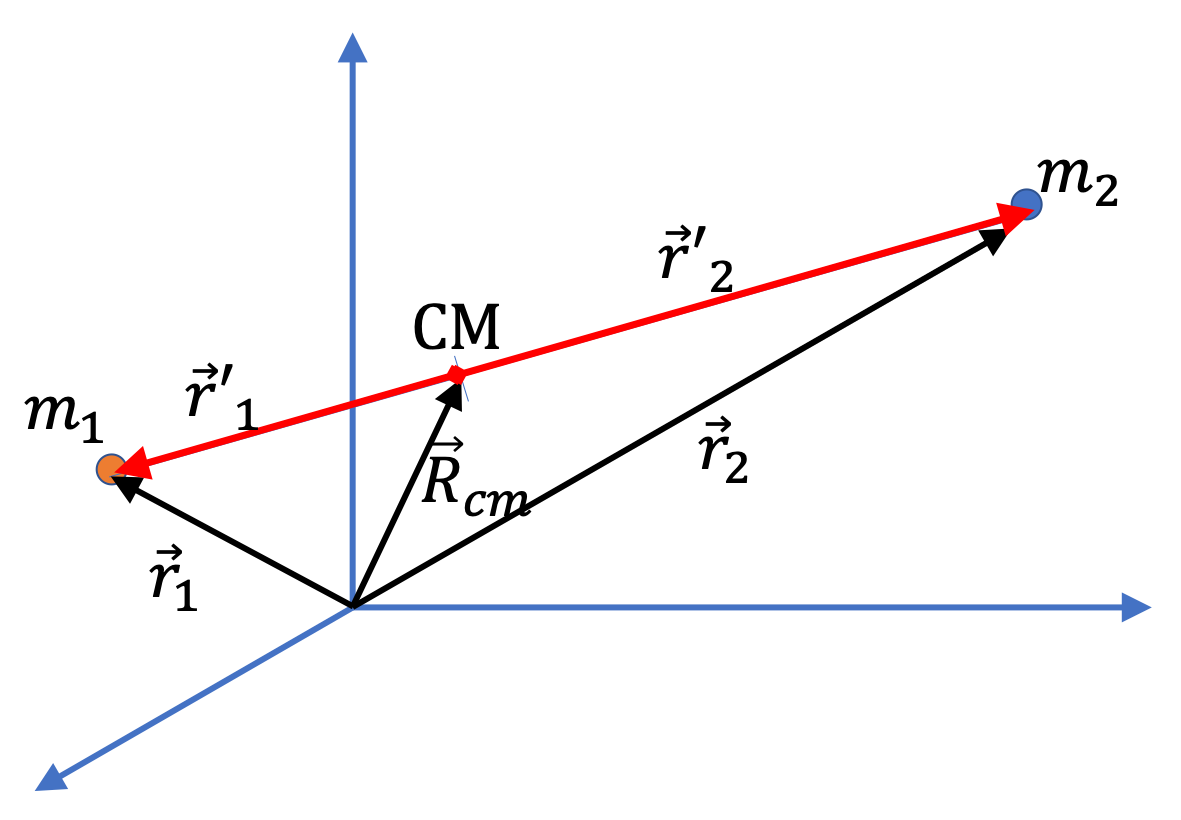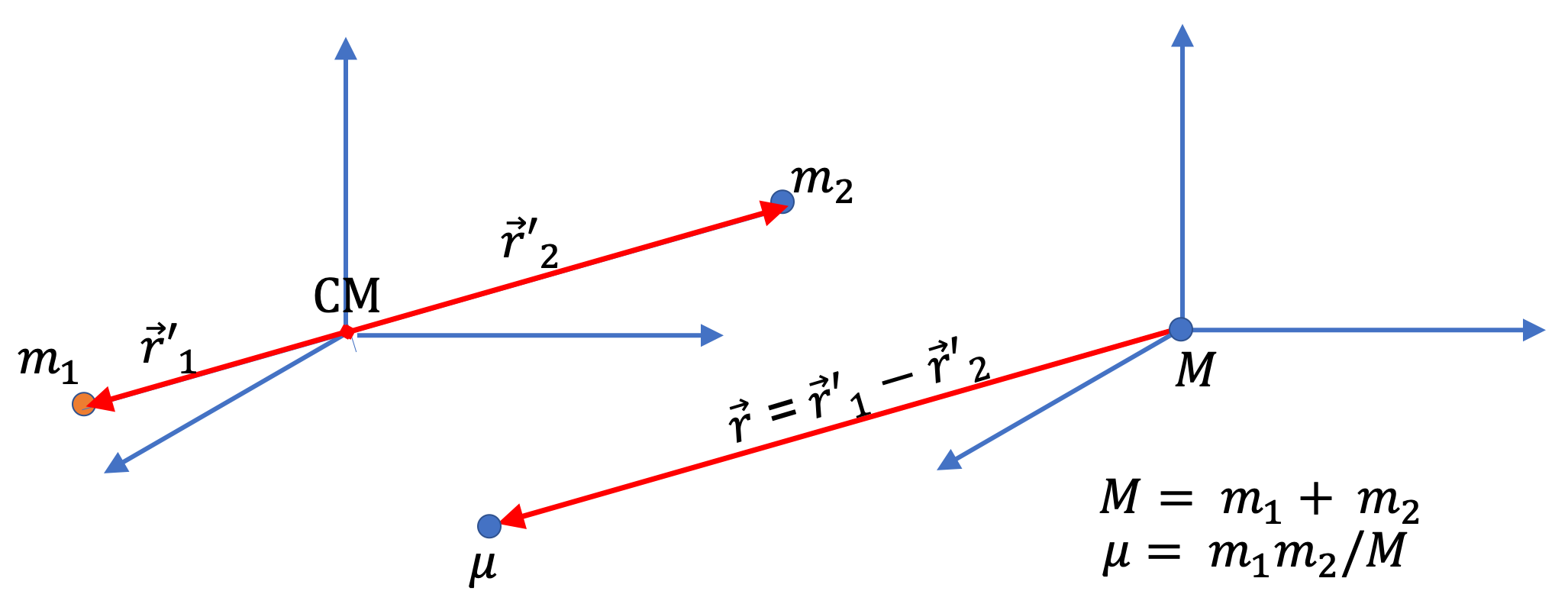## Section12.4The Two-Body Problem

We will consider motion of two bodies interacting with gravitational force only. We have seen before that motion separates into motion of the center of motion and relative position of the two bodies.

Let $m_1$ and $m_2$ be the masses and $\vec{r}_1$ and $\vec{r}_2$ their positions. Their separate equations of motions are

\begin{align} m_1 \vec{a}_1 \amp = G\dfrac{m_1m_2}{|\vec{r}_1 - \vec{r}_2|^2}\, \hat u_{1\rightarrow 2}, \label{eq-vectorized-law-of-grav-m1}\tag{12.4.1}\\ m_2 \vec{a}_2 \amp = -G\dfrac{m_1m_2}{|\vec{r}_1 - \vec{r}_2|^2}\, \hat u_{1\rightarrow 2}, \label{eq-vectorized-law-of-grav-m2}\tag{12.4.2} \end{align}

where $\hat u_{1\rightarrow 2}$ is a unit vector in the direction of $1\rightarrow 2\text{.}$ The unit vector is needed to express the direction of the vector equation analytically. Here, $| \vec{r}_1 - \vec{r}_2 |$ is the distance between the two bodies.

Now, from the sum and difference of Eqs. (12.4.1) and (12.4.2) we obtain two useful equations.

\begin{align} \amp \vec{A}_\text{cm} = 0, \label{eq-vectorized-law-of-grav-acm}\tag{12.4.3}\\ \amp m_1 \vec{a}_1 - m_2 \vec{a}_2 = 2G\dfrac{m_1m_2}{|\vec{r}_1 - \vec{r}_2|^2}\, \hat u_{1\rightarrow 2}, \label{eq-vectorized-law-of-grav-relative}\tag{12.4.4} \end{align}

The first of these equations, says that the CM has no acceleration. Therefore, if we use a coordinate system that moves with the CM, i.e., we let the origin to be at the CM. It turns out that this coordinate system also makes the second equation simpler. We say that we are using CM Frame. The coordinates are shown in Figure 12.4.1.Figure 12.4.1. The motion of two particles $m_1$ and $m_2$ interacting with gravitational force only are simpler if described with respect to the center of mass. The position vectors have the following relation: $\vec r_1 = \vec r^{\,\prime}_1 + \vec R_\text{cm}$ and $\vec r_2 = \vec r^{\,\prime}_2 + \vec R_\text{cm}\text{.}$

Let $\vec r^{\,\prime}_1$ $\vec r^{\,\prime}_2$ be the postions of the two particles with respect to their CM. Then, we have the following relations between the original coordinates and the new ones.

\begin{gather} \vec r^{\,\prime}_1 - \vec r^{\,\prime}_2 = \vec{r}_1 - \vec {r}_2, \label{eq-vectorized-law-of-grav-cm-coords-1}\tag{12.4.5}\\ m_1\vec r^{\,\prime}_1 + m_2 \vec r^{\,\prime}_2 = 0. \label{eq-vectorized-law-of-grav-cm-coords-2}\tag{12.4.6} \end{gather}

For convenience we give the relative position of particle 1 with respect to particle 2 its own symbol.

\begin{equation} \vec r = \vec r_1 - \vec r_2 = \vec r^{\,\prime}_1 - \vec r^{\,\prime}_2,\tag{12.4.7} \end{equation}

and acceleration of this coordinate, i.e., the relative acceleration,

\begin{equation} \vec a = \text{ acceleration of relative position } \vec r.\tag{12.4.8} \end{equation}

We also inroduce a combination of masses, called the reduced mass, denoted by $\mu\text{.}$

\begin{equation} \mu = \dfrac{m_1 m_2 }{m_1 + m_2},\tag{12.4.9} \end{equation}

and total mass by $M$

\begin{equation} M = m_1 + m_2.\tag{12.4.10} \end{equation}

After some algebra, which I differ to the end of this section, we can show that Eq. (12.4.4) gives the following for the magnitude of the relative acceleration,

\begin{equation} \mu\,\vec a = -G_N \dfrac{ M\,\mu}{r^2}\,\hat u,\label{eq-vectorized-law-of-grav-relative-cmframe}\tag{12.4.11} \end{equation}

where $\hat u$ is a unit vector pointed towards the origin.

That is, the original coupled systems of equation of the two-body system in (12.4.1) and (12.4.2), has been decoupled into two separate one-body problems: (1) Eq. (12.4.3) of the CM and (2) Eq. (12.4.11) for the relative coordinate in the center of frame.

If you were to look at the original two particles from CM, you would find their relative motion to be described by a single particle of mass $\mu$ moving in the field of a particle of mass $(m_1 + m_2)$ placed at the origin as illustrated in Figure 12.4.2. If $m_1\lt\lt m_2\text{,}$ then, the CM will be very near $m_2$ and $\mu\approx m_1\text{,}$ i.e., in this case, it would appear that $m_1$ is moving in the field of $m_2$ while $m_2$ is fixed, as is the case for Earth-Sun system.Figure 12.4.2. The problem of two particles $m_1$ and $m_2$ interacting with gravitational force only in CM frame becomes study of motion of one particle of mass $\mu$ moving in the gravitational field by an object of mass $M$ at the origin, which is at the CM.

Algebra for Equation (12.4.11)

\begin{align*} \vec a \amp = \vec a_1 - \vec a_2, \\ \amp = \left( \frac{1}{m_1} + \frac{1}{m_2} \right) G_N \frac{ m_1 m_2 }{r^2} \hat u_{1\rightarrow 2}. \end{align*}

Now, we write this in the CM frame (the right side of Figure 12.4.2), with $\hat u_{1\rightarrow 2}$ is radial unit vector $\hat u_{1\rightarrow 2}= \hat r = (\vec r)/r \text{.}$

\begin{equation*} \vec a = G_N \frac{ m_1 + m_2 }{r^2}\, \left( - \frac{\vec r}{r}\right). \end{equation*}

Now, multiplying by $\mu\text{,}$ we get

\begin{equation*} \mu\,\vec a = G_N \frac{ \mu M }{r^2}\, \hat u, \end{equation*}

where $\hat u = \frac{-\vec r}{r}$ is unit vector pointed towards the origin.